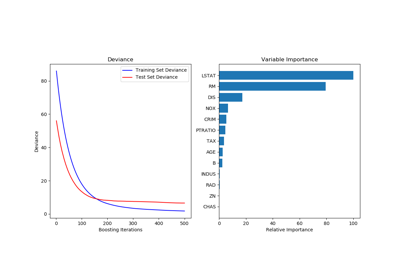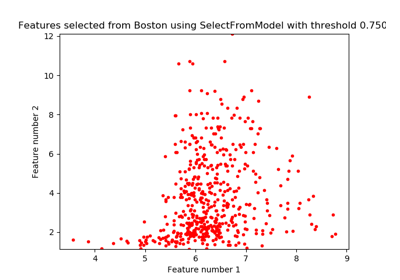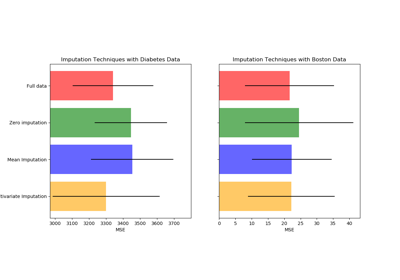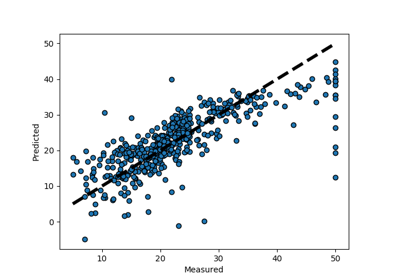# sklearn.datasets.load_boston¶

sklearn.datasets.load_boston(return_X_y=False)[source]

Load and return the boston house-prices dataset (regression).

 Samples total 506 Dimensionality 13 Features real, positive Targets real 5. - 50.

Read more in the User Guide.

Parameters: return_X_y : boolean, default=False. If True, returns (data, target) instead of a Bunch object. See below for more information about the data and target object. New in version 0.18. data : Bunch Dictionary-like object, the interesting attributes are: ‘data’, the data to learn, ‘target’, the regression targets, ‘DESCR’, the full description of the dataset, and ‘filename’, the physical location of boston csv dataset (added in version 0.20). (data, target) : tuple if return_X_y is True New in version 0.18.

Notes

Changed in version 0.20: Fixed a wrong data point at [445, 0].

Examples

>>> from sklearn.datasets import load_boston
>>> print(boston.data.shape)
(506, 13)


## Examples using sklearn.datasets.load_boston¶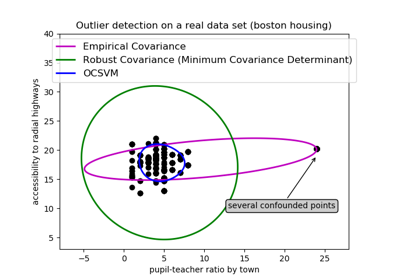Outlier detection on a real data set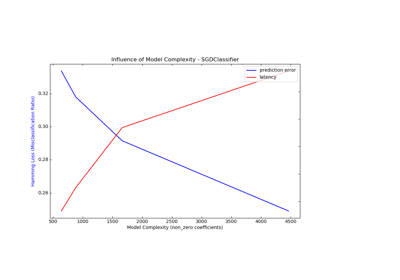Model Complexity Influence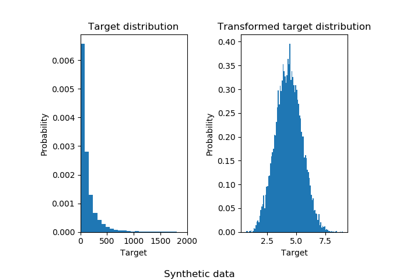Effect of transforming the targets in regression model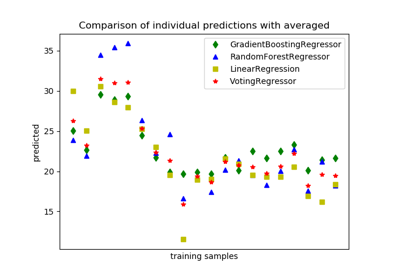Plot individual and voting regression predictions